# Entity Store of Books in Stephen Wolfram's Library

An entity store of books in Stephen Wolfram’s library that were used during in the creation of A New Kind of Science (Wolfram, 2002).

This is not Stephen Wolfram's complete library; only books used in one way or another for A New Kind of Science.

ISBNs for books were converted to OpenLibrary IDs, from which additional properties were deduced.

(1 entity types, 2611 entities, 9 properties)

## Examples

### Basic Examples

Retrieve the ResourceObject:

 In:=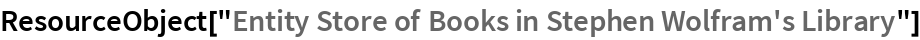Out=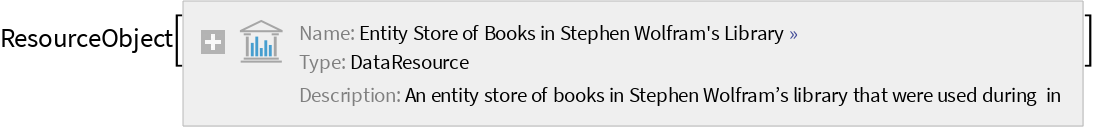View the data:

 In:=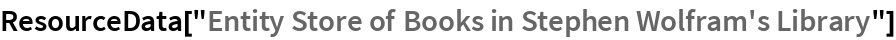Out=Register the EntityStore:

 In:=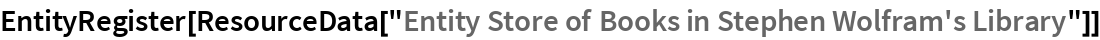Out=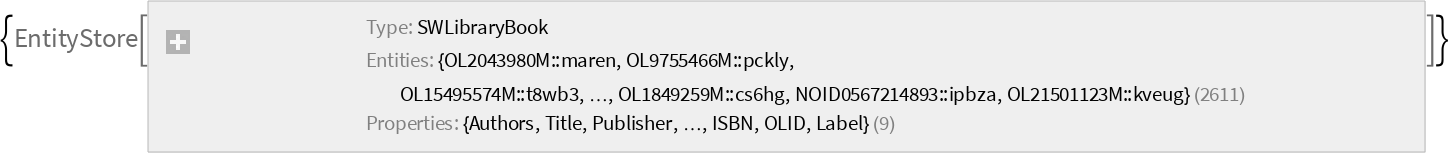### Analysis

Find the total number of books:

 In:=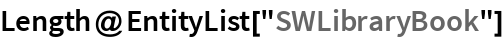Out=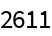Get the available properties for the entity store:

 In:=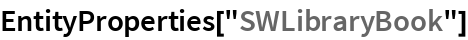Out=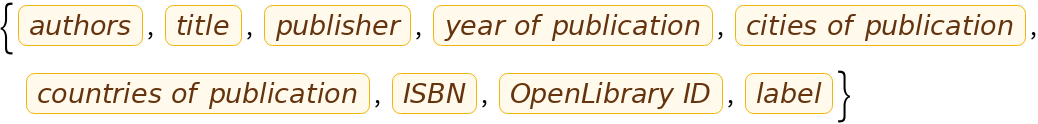Find the distribution of the books published after 1950:

 In:=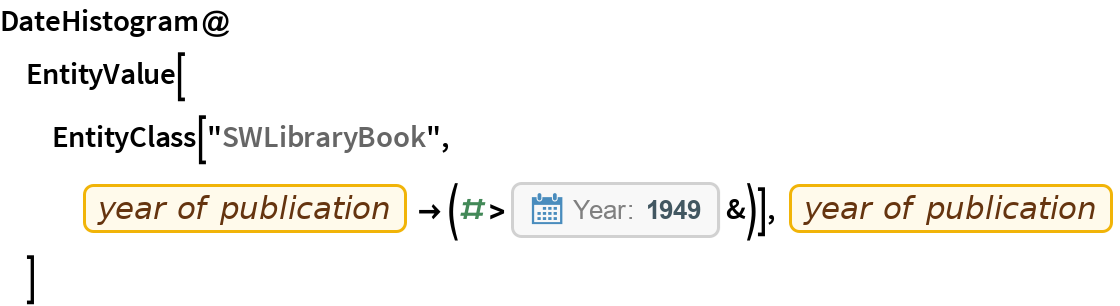Out=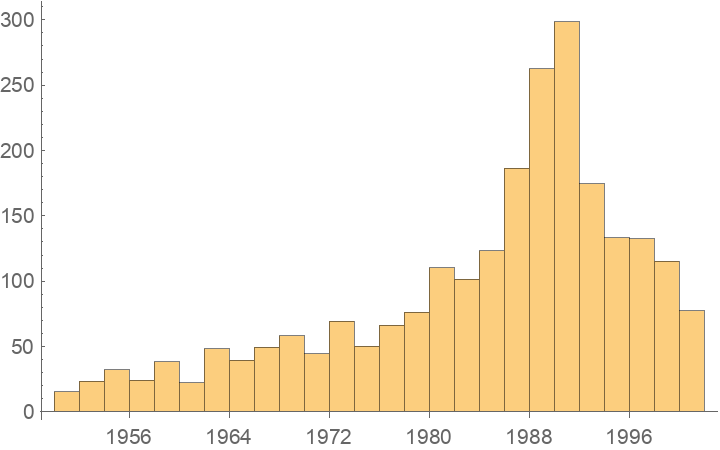Find the top 10 countries of publication:

 In:=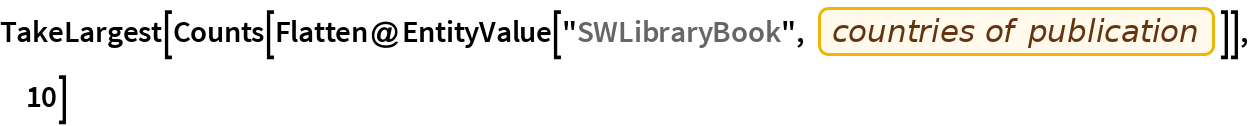Out=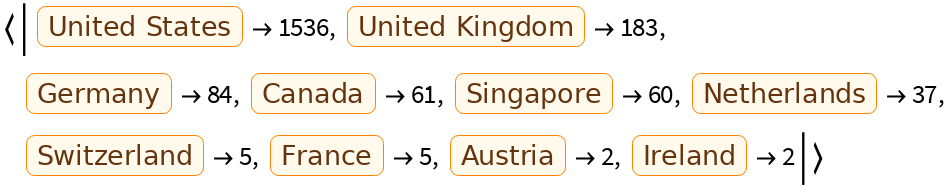Find books published in Ireland:

 In:=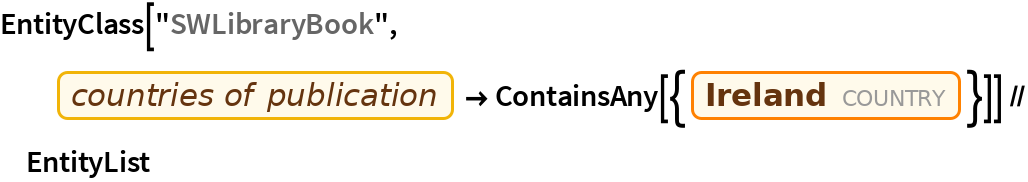Out=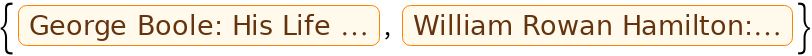Find countries with the smallest numbers of books published there:

 In:=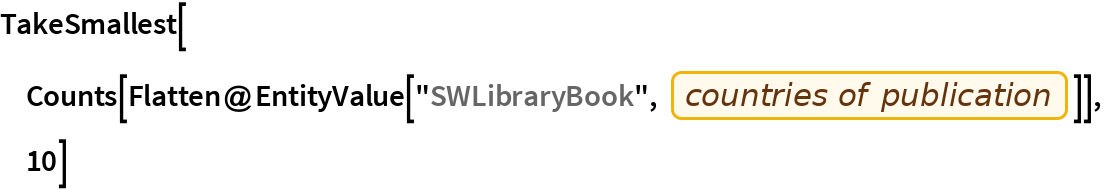Out=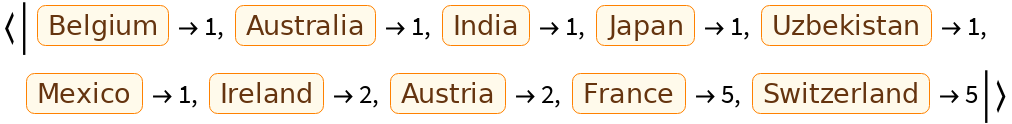Find the book published in Uzbekistan:

 In:=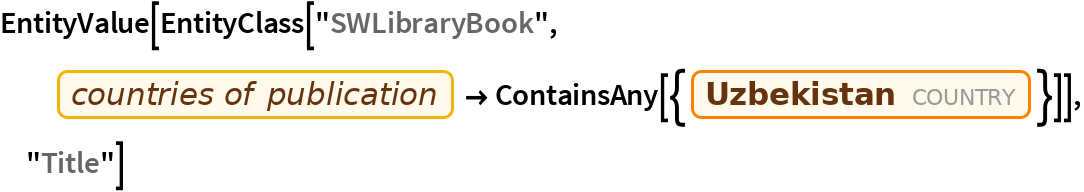Out=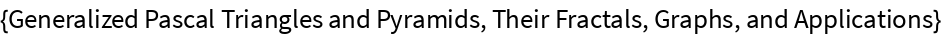Wolfram Research, "Entity Store of Books in Stephen Wolfram's Library" from the Wolfram Data Repository (2017)